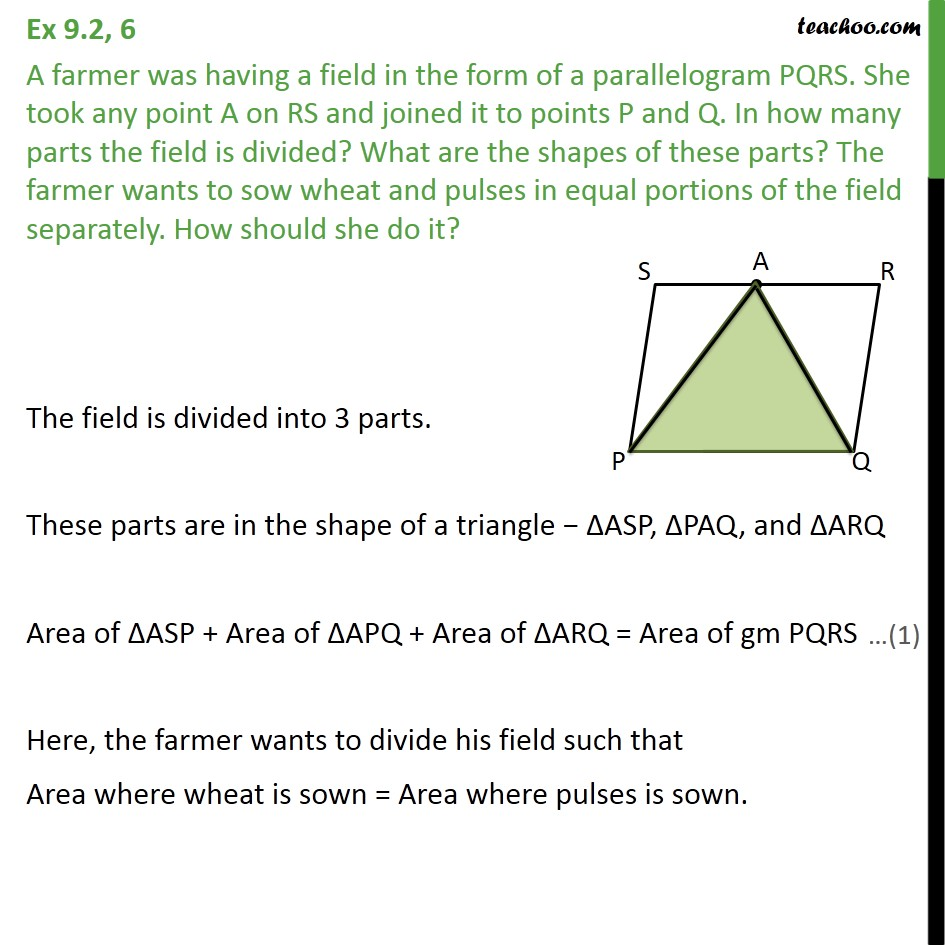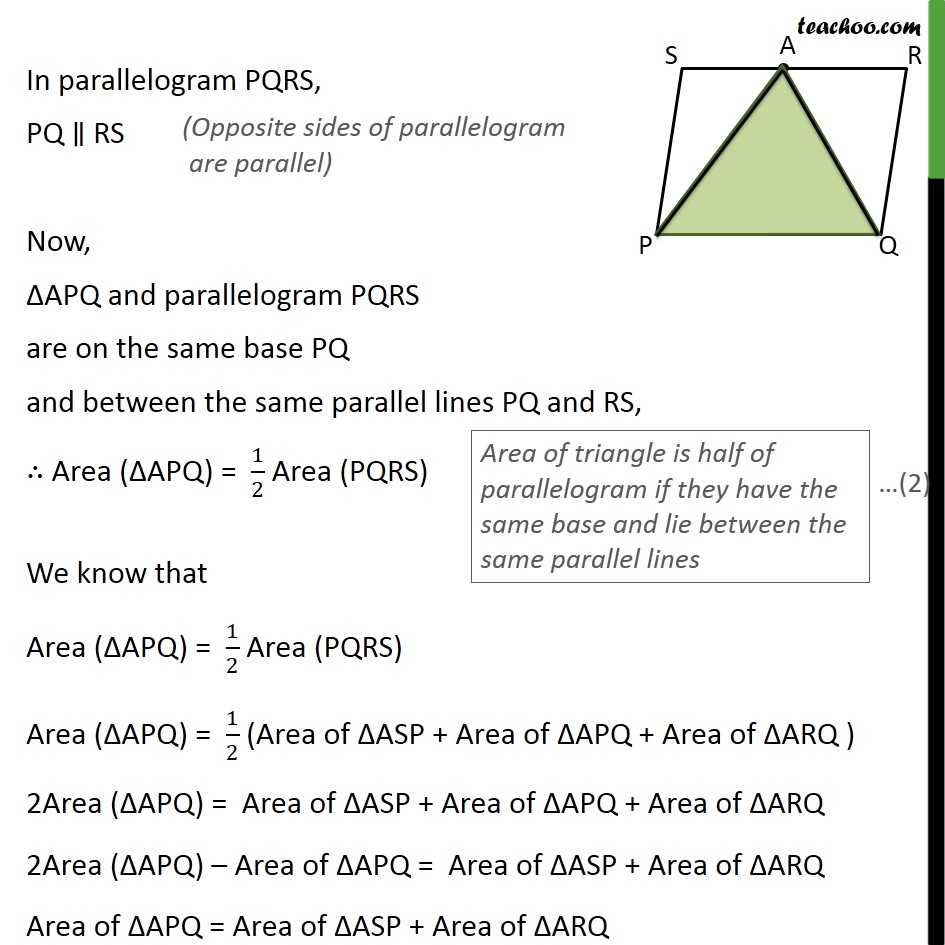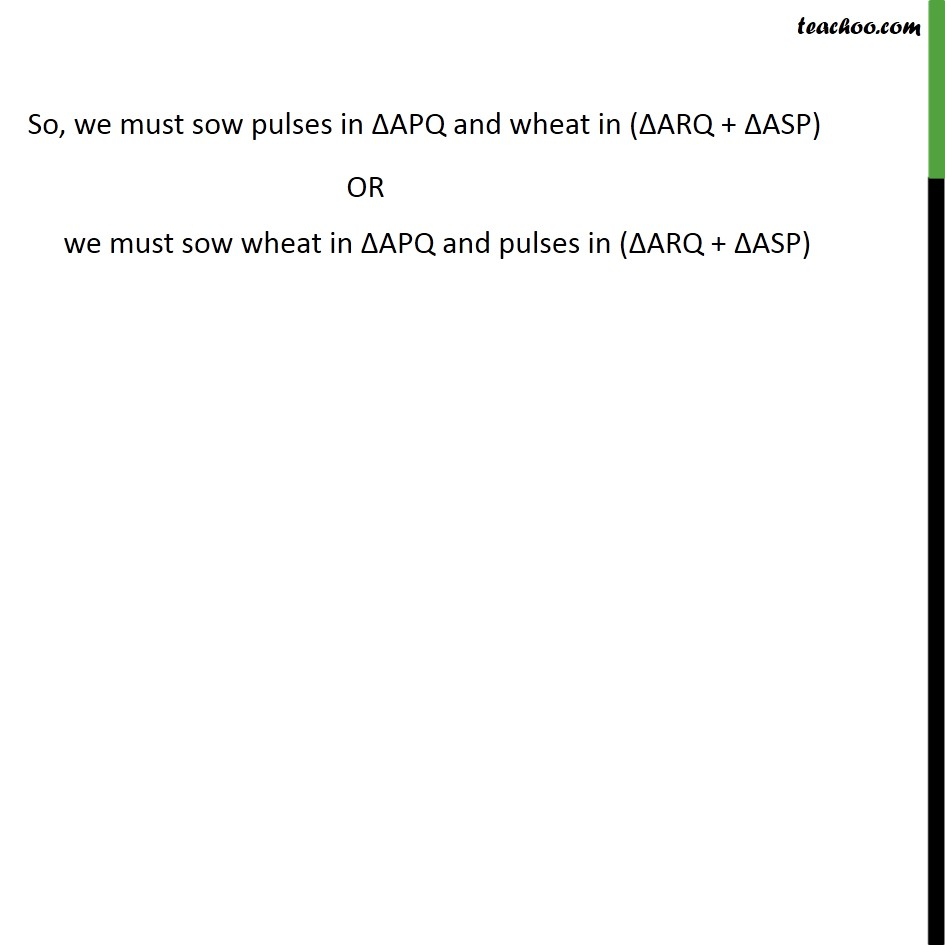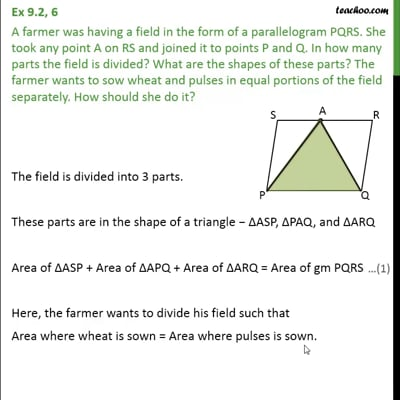Ex 9.2

Chapter 9 Class 9 - Areas of Parallelograms and Triangles [Deleted]
Serial order wiseThis video is only available for Teachoo black users

Solve all your doubts with Teachoo Black (new monthly pack available now!)

### Transcript

Ex 9.2, 6 A farmer was having a field in the form of a parallelogram PQRS. She took any point A on RS and joined it to points P and Q. In how many parts the field is divided? What are the shapes of these parts? The farmer wants to sow wheat and pulses in equal portions of the field separately. How should she do it? The field is divided into 3 parts. These parts are in the shape of a triangle − ΔASP, ΔPAQ, and ΔARQ Area of ΔASP + Area of ΔAPQ + Area of ΔARQ = Area of gm PQRS Here, the farmer wants to divide his field such that Area where wheat is sown = Area where pulses is sown. In parallelogram PQRS, PQ ∥ RS Now, ΔAPQ and parallelogram PQRS are on the same base PQ and between the same parallel lines PQ and RS, ∴ Area (ΔAPQ) = 1/2 Area (PQRS) We know that Area (ΔAPQ) = 1/2 Area (PQRS) Area (ΔAPQ) = 1/2 (Area of ΔASP + Area of ΔAPQ + Area of ΔARQ ) 2Area (ΔAPQ) = Area of ΔASP + Area of ΔAPQ + Area of ΔARQ 2Area (ΔAPQ) – Area of ΔAPQ = Area of ΔASP + Area of ΔARQ Area of ΔAPQ = Area of ΔASP + Area of ΔARQ So, we must sow pulses in ΔAPQ and wheat in (ΔARQ + ΔASP) OR we must sow wheat in ΔAPQ and pulses in (ΔARQ + ΔASP)